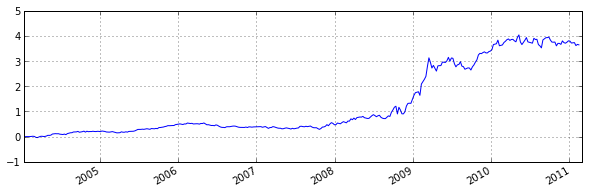In :
import pandas as pd
import pandas.io.data
import numpy as np

delta_t = 3


Load data: Dow Jones Industrial Average, S&P500 from yahoo and the google trend weekly averages.

In :
djia = pd.DataFrame.from_csv('DJIA.csv', index_col='DATE').sort()
sp500 = pd.DataFrame(pd.io.data.DataReader("^GSPC", "yahoo", start=datetime.datetime(2004, 1, 1), end=datetime.datetime(2011, 4, 1))['Adj Close'], columns=['sp500'])
#sp500 = sp500.tshift(1, freq='B')
dija.index = dija.index.to_datetime()
djia.rename(columns={'VALUE': 'djia'}, inplace=True)
debt.index = debt.index.to_datetime()
debt = debt.tshift(2, freq='D') # Moves Saturday to Monday

columns = ['Google Start Date', 'Google End Date', 'debt', 'DJIA Date', 'DJIA Closing Price']
df = df[columns]
debt_from_study = df[['debt', 'DJIA Closing Price']]
debt_from_study.rename(columns={'debt': 'debt_preis', 'DJIA Closing Price': 'djia_preis'}, inplace=True)
debt_from_study.index = debt_from_study.index.to_datetime()
debt_from_study = debt_from_study.tshift(2, freq='D') # Move from Sat to Monday

Out:
debt_preis djia_preis
2004-01-12 0.210000 10485.18
2004-01-19 0.210000 10528.66
2004-01-26 0.210000 10702.51
2004-02-02 0.213333 10499.18
2004-02-09 0.200000 10579.03
In :
# Join together into single dataframe.
data = pd.concat([debt, sp500, debt_from_study], axis=1).dropna()

Out:
debt sp500 debt_preis djia_preis
2004-01-12 35 1127.23 0.210000 10485.18
2004-01-26 36 1155.37 0.210000 10702.51
2004-02-02 37 1135.26 0.213333 10499.18
2004-02-09 37 1139.81 0.200000 10579.03
2004-02-23 35 1140.99 0.200000 10609.62
In :
data.debt.plot()
data.debt_preis.plot(secondary_y=True)

Out:
<matplotlib.axes.AxesSubplot at 0xa6c546c>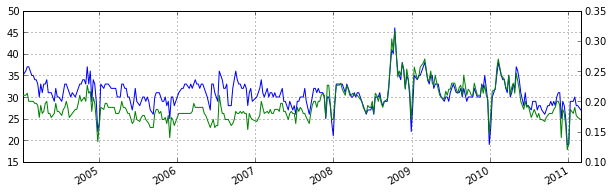In :
data['rolling_mean'] = pd.rolling_mean(data.debt, delta_t).shift(1)
data['rolling_mean_preis'] = pd.rolling_mean(data.debt_preis, delta_t).shift(1)

Out:
<class 'pandas.core.frame.DataFrame'>
DatetimeIndex: 10 entries, 2004-01-12 00:00:00 to 2004-03-29 00:00:00
Data columns (total 11 columns):
debt                    10  non-null values
sp500                   10  non-null values
debt_preis              10  non-null values
djia_preis              10  non-null values
rolling_mean            7  non-null values
rolling_mean_preis      7  non-null values
order                   10  non-null values
order_preis             10  non-null values
log_returns             10  non-null values
log_returns_sp          10  non-null values
log_returns_new_data    10  non-null values
dtypes: float64(9), int64(2)


In :
data['order'] = 0
data['order'][data.debt > data.rolling_mean.shift(1)] = -1 # Shorting if cross-over
data['order'][data.debt < data.rolling_mean.shift(1)] = 1 # Buying otherwise.
data['order'].ix[:delta_t] = 0

data['order_preis'] = 1
data['order_preis'][data.debt_preis > data.rolling_mean_preis] = -1 # Shorting if cross-over
data['order_preis'][data.debt_preis < data.rolling_mean_preis] = 1 # Buying otherwise.
data['order_preis'].ix[:delta_t] = 0

In :
print "% of overlapping trading signals from Preis debt data and newly retrieved search data: ", (data.order == data.order_preis).mean()

% of overlapping trading signals from Preis debt data and newly retrieved search data:  0.72619047619

In :
data.head(10)

Out:
<class 'pandas.core.frame.DataFrame'>
DatetimeIndex: 10 entries, 2004-01-12 00:00:00 to 2004-03-29 00:00:00
Data columns (total 11 columns):
debt                    10  non-null values
sp500                   10  non-null values
debt_preis              10  non-null values
djia_preis              10  non-null values
rolling_mean            7  non-null values
rolling_mean_preis      7  non-null values
order                   10  non-null values
order_preis             10  non-null values
log_returns             10  non-null values
log_returns_sp          10  non-null values
log_returns_new_data    10  non-null values
dtypes: float64(9), int64(2)


From the paper: "If we take a ‘short position’—selling at the closing price p(t) and buying back at price p(t + 1)—then the cumulative return R changes by log(p(t)) − log(p(t + 1)). If we take a ‘long position’—buying at the closing price p(t) and selling at price p(t + 1)—then the cumulative return R changes by log(p(t + 1)) − log(p(t))."

In :
# Compute log returns using djia and debt search volume from Preis et al.
data['log_returns'] = data.order_preis * np.log(data.djia_preis.shift(-1)) - data.order_preis * np.log(data.djia_preis)
# Compute log returns using S&P500.
data['log_returns_sp'] = data.order_preis * np.log(data.sp500.shift(-1)) - data.order_preis * np.log(data.sp500)
# Compute log returns using debt search volume recently extracted from trends
data['log_returns_new_data'] = data.order * np.log(data.djia_preis.shift(-1)) - data.order * np.log(data.djia_preis)

In :
plt.figsize(10, 3)
(np.exp(data.log_returns.cumsum()) - 1).plot();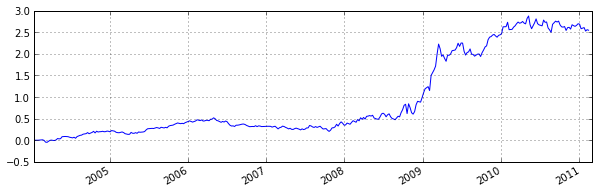In :
from IPython.core.display import Image
Image("http://www.nature.com/srep/2013/130425/srep01684/carousel/srep01684-f2.jpg")

Out: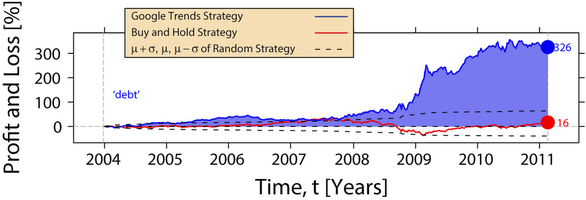Pretty good replication (using same data).

Lets see how well the strategy does on search volume data extracted more recently.

In :
plt.figsize(10, 3)
(np.exp(data.log_returns_new_data.cumsum()) - 1).plot();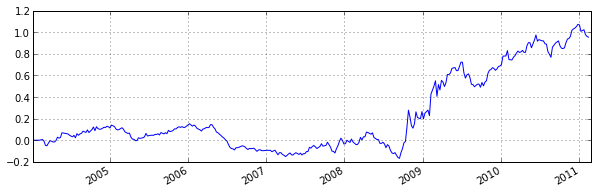Much worse. As seen before there is a qualitative difference between the search data used in Preis and the one that can be downloaded today. This might be expected since the signal is indeed degraded as you mentioned (only 72% of the order decisions match when comparing the two data sources).

Finally, lets see how well the strategy (using the Preis signals) would do on S&P500.

In :
plt.figsize(10, 3)
(np.exp(data.log_returns_sp.cumsum()) - 1).plot();